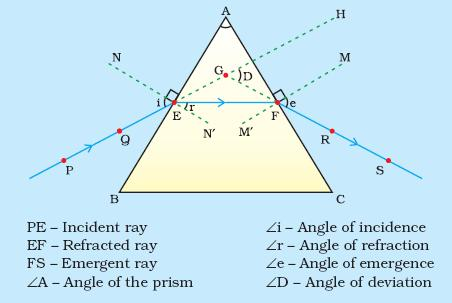# The refractive index of the material of a prism is   and the angle of the prism is 30o. One of the two refracting surfaces of the prism is made a mirror inwards, by silver coating. A beam of monochromatic light entering the prism from the other face will retrace its path (after reflection from the silvered surface) if its angle of incidence on the prism is Option 1) 30o Option 2) 45o Option 3) 60o Option 4) zero

P Plabita

As we have learned

Angle of deviation through prism -- wherein

angle of incidence

angle of emergence

angle of prismOption 1)

30o

This is incorrect

Option 2)

45o

This is correct

Option 3)

60o

This is incorrect

Option 4)

zero

This is incorrect

Exams
Articles
Questions